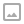·   ·  ☕ 1 min read

void mainImage( out vec4 fragColor, in vec2 fragCoord )
{
// Calculate coordinate with 0,0 at middle of screen
vec2 uv = (fragCoord - 0.5 * iResolution.xy) / iResolution.y;
// distance in UV Space from center of screen to current fragment
float distance = length(uv);
// Calculate the angle to the current fragement from the center of screen
float angle = atan(uv.y, uv.x);

float pi2 = 3.14159265 * 2.0;
// Individual color functions combined into a single line:
// float r = sin(distance * 50.0 + angle + iTime);
// float g = sin(distance * 50.0 + angle + iTime + (1.0 / 3.0) * pi2);
// float b = sin(distance * 50.0 + angle + iTime + (2.0 / 3.0) * pi2);
// Condense the above into this:
fragColor = sin(distance * 50.0 + angle + iTime + (vec4(0.0,1.0,2.0,0.0) * pi2 / 3.0));

fragColor = clamp(fragColor, 0.0, distance); // Fade colors towards center
}


I also recorded a walkthrough of building this shader that goes into a bit more depth about how everything works. You can see it on YouTube.WRITTEN BY
Sam Wronski
Maker of things, currently helping build cloud things @ Google. World of Zero is a personal project disconnected from my professional work. Lets make something awesome together!